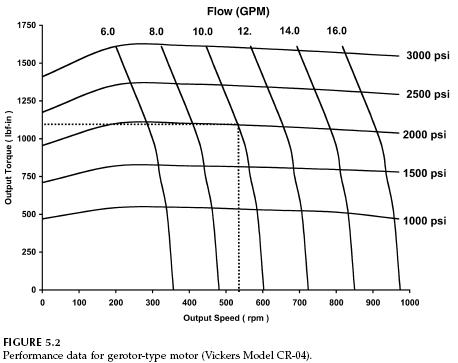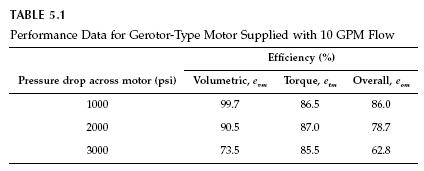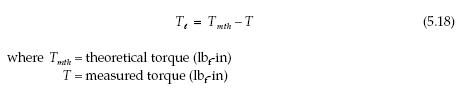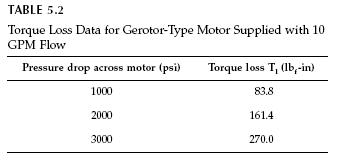﻿ Hydraulic Motor: Typical Performance Data for a Hydraulic Gear Motor | Hydraulic Schematic Troubleshooting

Typical Performance Data for a Hydraulic Gear Motor

Manufacturer’s data for a gear motor are given in Fig. 5.2. These data serve as an example of typical data supplied by a manufacturer. The sloping horizontal curves are pressure drop (?P across motor), and the sloping vertical curves are flow to the motor. The vertical grid lines are output speed, and the horizontal grid lines are output torque. The operating point for the previous example (10 GPM, 2000 psi) is shown in the figure.Data from Fig. 5.2, published by the manufacturer, was used to calculate the efficiencies given in Table 5.1. The 10 GPM curve was used for all three operating points, 1000, 2000, and 3000 psi. The volumetric and overall efficiencies follow the same trend seen for the gear pump.Leakage increases as pressure increases; consequently, efficiency decreases. For this particular design of gear motor, the efficiency decreases significantly when pressure increases above 2000 psi. A secondary effect is the deformation of the components. Clearance between parts increases with pressure, thus the effective area of the leakage pathway increases.

Torque loss is defined byThe torque loss at the various load pressures is given in Table 5.2. Since torque loss for this motor design is approximately a linear function of pressure, the torque efficiency is approximately constant (Table 5.1).Categories: Hydraulic Motor | Tags: , | Leave a comment